# OpenCV development notes (sixty): The red fat man takes you in 8 minutes to learn more about Harris corner detection (graphics + easy to understand + program source code)

Posted May 26, 20208 min read

If the article is an original article, it may not be reprinted without permission
Dear readers, knowledge is endless and manpower is poor, either change the demand, or find a professional, or research by yourself
Red Fat Man(Red Imitation) blog post Daquan:Development technology collection(including Qt practical technology, Raspberry Pi, 3D, OpenCV, OpenGL, ffmpeg, OSG, microcontroller, soft and hard combination, etc.) Continuous update ...(click on the portal)

OpenCV development column(click on the portal)
================================================== ================================================== ======================

# Foreword

Red fat man, come too!
Recognition, sometimes meet the needs, such as identifying a triangle, and find the angle of the three vertices of the triangle, this is similar to the educational scene, there are other scenes, then it is very important to detect the corner point, the angle is detected Point and find its angle.

# Demo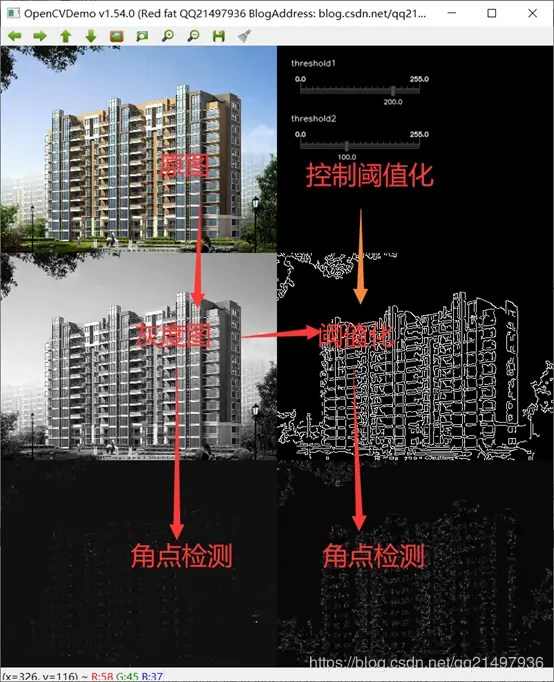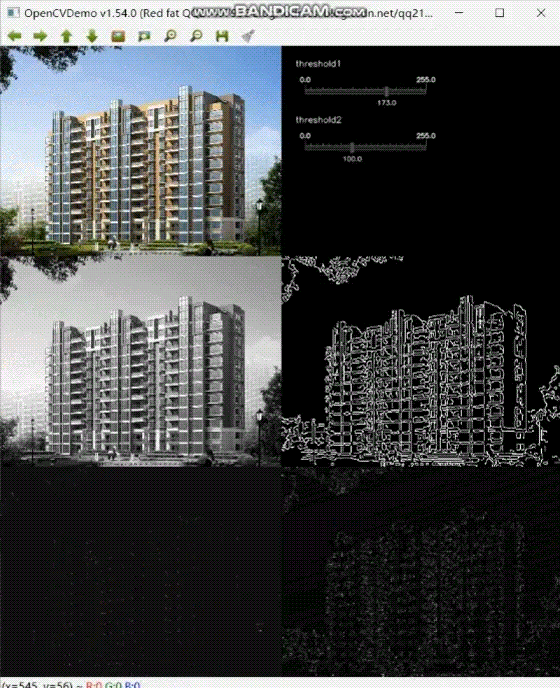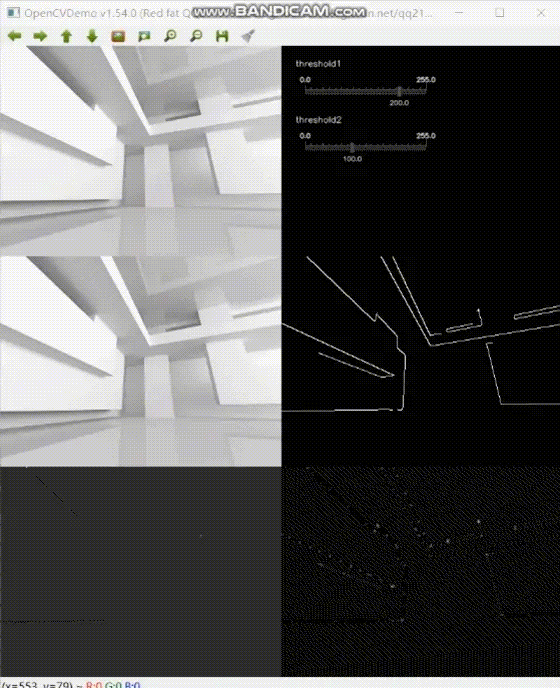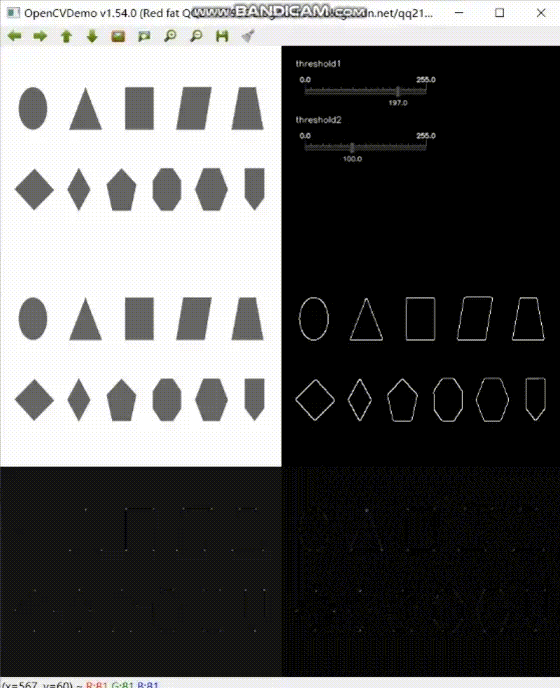# Three types of image features

• Edge:the area where the image intensity changes suddenly is actually a high intensity gradient area;
• Corner:where the two edges meet, it looks like a corner;
• Spots:areas divided by features, areas with particularly high intensity, particularly low intensity, or with specific textures;

# Harris corner

## Overview

Harris corner detection is a corner extraction algorithm based on gray-scale image, with high stability. The performance of harris corner detection in opencv is relatively low because it uses Gaussian filtering.
Gray image-based corner detection is divided into gradient-based, template-based and template-gradient-based combination of three types of methods, and Harris algorithm is based on the gray-scale image based on the template type algorithm.

## principle

The identification of the corner points of the human eye is usually done in a local small window:if the small window is moved in various directions, the grayscale in the window changes greatly, then there is a corner point in the window. Divided into the following three situations:

• If moving in all directions, the gray level is almost unchanged, indicating a flat area;
• If it only moves in a certain direction, the gray level is almost unchanged, indicating a straight line;
• If you move in all directions, the gray scale changes, indicating that it is a corner.

The basic principle is as follows:The specific calculation formula is as follows:Taylor expands: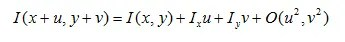Substitute to get: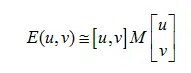among them:The quadratic function is essentially an ellipse function. The flatness and size of the ellipse are determined by the two eigenvalues of the matrix M.The relationship between the two eigenvalues of matrix M and the corners, edges, and flat areas in the image.
Harris defines the corner response function as: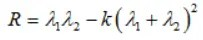R = Det(M) -k_trace(M) _trace(M), k is the empirical constant 0.04 ~ 0.06.
Defined as the point when R> threshold and the local maximum value is defined as the corner point.

## Harris function prototype

``````void cornerHarris(InputArray src,
OutputArray dst,
int blockSize,
int ksize,
double k,
intborderType = BORDER DEFAULT);``````
• Parameter one:InputArray type src, the input image, that is, the source image, just fill the Mat class object, and it must be a single channel 8-bit or floating-point image;
• Parameter 2:dst of OutputArray type, the operation result after the function call exists here, that is, this parameter is used to store the output result of Harris corner detection, and it has the same size as the source image. Pay special attention to the output type is CV \ _32F;
• Parameter 3:blockSize of int type, indicating the size of the neighborhood;
• Parameter four:ksize of int type, indicating the aperture size of Sobel() operator;
• Parameter 5:Double type k, Harris corner response function, generally 0.04 ~ 0.06;
• Parameter 6:borderType of int type, border mode of image pixels: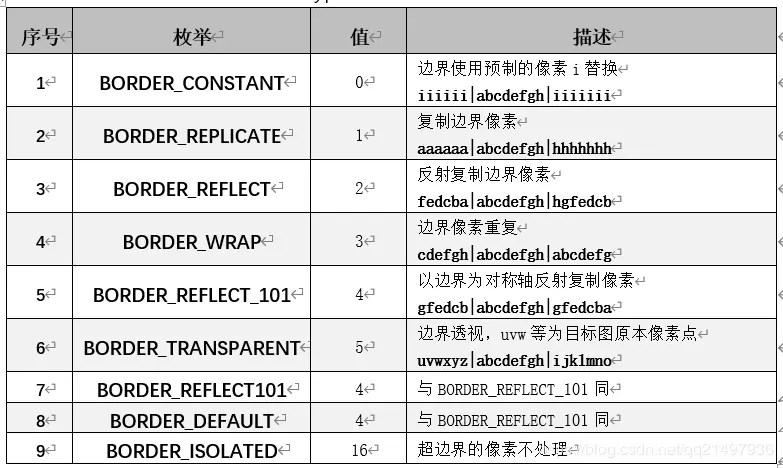## Normalization overview

Normalization refers to the normalization operation of matrix cv ::Mat.
Normalization is a dimensionless processing method, which makes the absolute value of the physical system value into some relative value relationship. An effective way to simplify calculations and reduce magnitude. For example, after the frequency values in the filter are normalized to the cutoff frequency, the frequencies are all relative values of the cutoff frequency, and there is no dimension. After the impedance is normalized to the internal resistance of the power supply, each impedance becomes a relative impedance value, and the dimension "ohm" is gone. After the various operations are completed, everything is restored by denormalization. The nyquist frequency is often used in the signal processing toolbox. It is defined as one-half of the sampling frequency. Both the order selection of the filter and the cut-off frequency in the design are normalized using the nyquist frequency. For example, for a system with a sampling frequency of 500hz, the normalized frequency of 400hz is 400/500 = 0.8, and the normalized frequency range is between \ [0,1 ].

## Normalized function prototype

``````void normalize(InputArray src,
InputOutputArray dst,
double alpha = 1,
double beta = 0,
int norm_type = NORM_L2,
int dtype = -1,
• Parameter one:InputArray type src, generally mat;
• Parameter 2:Input output array type dst, generally mat, the same size as src;
• Parameter III:double type alpha, normalized minimum value, default value 1;
• Parameter 4:double type of beta, the normalized maximum value, the default value is 0;
• Parameter 5:norm \ _type of int type, normalized type, see cv ::NormTypes for details, the default is;
• Parameter 6:dtype of int type, default value -1, when negative, its output matrix is the same as src type, otherwise it has the same number of channels as src, and the image depth is CV \ _MAT \ _DEPTH.
• Parameter 7:InputArray type mask, optional operation mask, the default value is noArray();

## Enhanced image function prototype

``````void convertScaleAbs(InputArray src,
OutputArray dst,
double alpha = 1,
double beta = 0);``````
• Parameter one:InputArray type src, generally mat;
• Parameter 2:Output array type dst, generally mat, the size is the same as src;
• Parameter 3:double type alpha, normalized maximum value, default value 1;
• Parameter 4:double type of beta, the normalized maximum value, the default value is 0;

# Demo source code

``````void OpenCVManager ::testHarris()
{
QString fileName1 =
"E:/qtProject/openCVDemo/openCVDemo/modules/openCVManager/images/16.jpg";
int width = 400;
int height = 300;

cv ::Mat srcMat = cv ::imread(fileName1.toStdString());
cv ::resize(srcMat, srcMat, cv ::Size(width, height));

cv ::String windowName = _windowTitle.toStdString();
cvui ::init(windowName);

cv ::Mat windowMat = cv ::Mat(cv ::Size(srcMat.cols * 2, srcMat.rows * 3),
srcMat.type());

int threshold1 = 200;
int threshold2 = 100;
while(true)
{
windowMat = cv ::Scalar(0, 0, 0);

cv ::Mat mat;

cv ::Mat tempMat;
//Copy the original image to the left first
mat = windowMat(cv ::Range(srcMat.rows * 0, srcMat.rows * 1),
cv ::Range(srcMat.cols * 0, srcMat.cols * 1));
cv ::addWeighted(mat, 0.0f, srcMat, 1.0f, 0.0f, mat);

{
//grayscale image
cv ::Mat grayMat;
cv ::cvtColor(srcMat, grayMat, cv ::COLOR_BGR2GRAY);
//copy
mat = windowMat(cv ::Range(srcMat.rows * 1, srcMat.rows * 2),
cv ::Range(srcMat.cols * 0, srcMat.cols * 1));
cv ::Mat grayMat2;
cv ::cvtColor(grayMat, grayMat2, cv ::COLOR_GRAY2BGR);
cv ::addWeighted(mat, 0.0f, grayMat2, 1.0f, 0.0f, mat);

//Mean filter
cv ::blur(grayMat, tempMat, cv ::Size(3, 3));

cvui ::printf(windowMat, width * 1 + 20, height * 0 + 20, "threshold1");
cvui ::trackbar(windowMat, width * 1 + 20, height * 0 + 40, 200, & threshold1, 0, 255);
cvui ::printf(windowMat, width * 1 + 20, height * 0 + 100, "threshold2");
cvui ::trackbar(windowMat, width * 1 + 20, height * 0 + 120, 200, & threshold2, 0, 255);

//canny edge detection
cv ::Canny(tempMat, tempMat, threshold1, threshold2);
//copy
mat = windowMat(cv ::Range(srcMat.rows * 1, srcMat.rows * 2),
cv ::Range(srcMat.cols * 1, srcMat.cols * 2));
cv ::cvtColor(tempMat, grayMat2, cv ::COLOR_GRAY2BGR);
cv ::addWeighted(mat, 0.0f, grayMat2, 1.0f, 0.0f, mat);

//harris corner detection
cv ::cornerHarris(grayMat, grayMat2, 2, 3, 0.01);
//Normalization and conversion
cv ::normalize(grayMat2, grayMat2, 0, 255, cv ::NORM_MINMAX, CV_32FC1, cv ::Mat());
cv ::convertScaleAbs(grayMat2, grayMat2); //Linearly transform the normalized graph to 8U bit sign integer
//copy
mat = windowMat(cv ::Range(srcMat.rows * 2, srcMat.rows * 3),
cv ::Range(srcMat.cols * 0, srcMat.cols * 1));
cv ::cvtColor(grayMat2, grayMat2, cv ::COLOR_GRAY2BGR);

cv ::addWeighted(mat, 0.0f, grayMat2, 1.0f, 0.0f, mat);

//harris corner detection
cv ::cornerHarris(tempMat, tempMat, 2, 3, 0.01);
//Normalization and conversion
cv ::normalize(tempMat, tempMat, 0, 255, cv ::NORM_MINMAX, CV_32FC1, cv ::Mat());
cv ::convertScaleAbs(tempMat, tempMat); //Linearly transform the normalized graph to 8U bit sign integer
//copy
mat = windowMat(cv ::Range(srcMat.rows * 2, srcMat.rows * 3),
cv ::Range(srcMat.cols * 1, srcMat.cols * 2));
cv ::cvtColor(tempMat, tempMat, cv ::COLOR_GRAY2BGR);
cv ::addWeighted(mat, 0.0f, tempMat, 1.0f, 0.0f, mat);
}
//update
cvui ::update();
//show
cv ::imshow(windowName, windowMat);
//Esc key to exit
if(cv ::waitKey(25) == 27)
{
break;
}
}
}``````

# Engineering template:corresponding version number v1.54.0

Corresponding version number v1.54.0